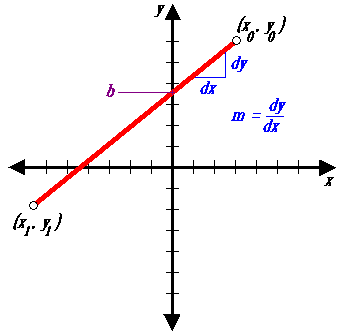# Simple Line

##```public void lineSimple(int x0, int y0, int x1, int y1, Color color) {
int pix = color.getRGB();
int dx = x1 - x0;
int dy = y1 - y0;
raster.setPixel(pix, x0, y0);
if (dx != 0) {
float m = (float) dy / (float) dx;
float b = y0 - m*x0;
dx = (x1 > x0) ? 1 : -1;
while (x0 != x1) {
x0 += dx;
y0 = Math.round(m*x0 + b);
raster.setPixel(pix, x0, y0);
}
}
}

```

The first line-drawing algorithm presented is called the simple slope-intercept algorithm. It is a straight forward implementation of the slope-intercept formula for a line.

 Web www.vrarchitect.net